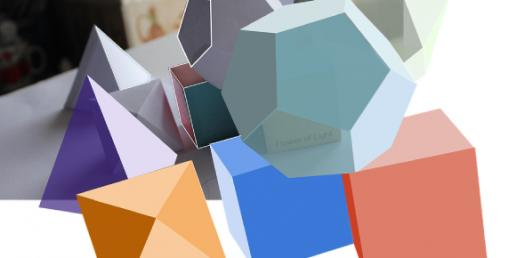# Can You Pass This Polyhedron Quiz?

12 Questions | Total Attempts: 3166SettingsThis quiz covers the basic topics involved in Platonic solids and other polyhedra. It is intended for introductory high school geometry and does not cover angles or trigonometry. Read the questions carefully and answer. So, let's try out the quiz. All the best!

• 1.
Another name for a corner, or point, is
• A.

Face

• B.

Edge

• C.

Vertex

• D.

Polygon

• 2.
On a cube, there are a total of
• A.

8 faces and 6 edges

• B.

6 faces and 8 edges

• C.

6 faces and 12 edges

• D.

6 faces and 16 edges

• 3.
Every polygon must have the same number of
• A.

Edges as vertices

• B.

Faces as vertices

• C.

Edges as faces

• D.

None of the above

• 4.
If you truncate one vertex of the dodecahedron shown, the new face created will be a
• A.

Triangle

• B.

Square

• C.

Pentagon

• D.

Octagon

• 5.
How many Platonic Solids are there?
• A.

Four

• B.

Five

• C.

Six

• D.

Seven

• 6.
Which of the following is NOT a Platonic Solid?
• A.

Tetrahedron

• B.

Octahedron

• C.

Rhombohedron

• D.

Icosahedron

• 7.
In the topic of polyhedrons and polygons, "regular" means
• A.

They are shaped correctly

• B.

They have more than six faces

• C.

All edges are equal length

• D.

All faces are equilateral triangles

• 8.
The shape in the diagram below is not a regular polyhedron because it has
• A.

More than four faces

• B.

Faces that are different shapes

• C.

Edge angles less than 60 degrees

• D.

Edges that are all the same length

• 9.
An architect want to make a skylight which has the shape of an icosahedron. She will need panes of glass which are
• A.

Triangular

• B.

Various sizes

• C.

Square

• D.

Hexagonal

• 10.
Which of the following shapes can NOT be fit together to make a regular polyhedron?
• A.

Octagon

• B.

Pentagon

• C.

Square

• D.

Triangle

• 11.
The prefix "poly" means
• A.

Bird

• B.

Plastic

• C.

Many

• D.

Shape

• 12.
What is another name for a cube?
• A.

Tetrahedron

• B.

Hexahedron

• C.

Octahedron

• D.

Decahedron

Related TopicsBack to top